In the course of training in programming in Visual Basic, Delphi, Java or C++ trainees are usually offered to write as the first program the program "Hello Word". It is possibly right. As a rule you need only 2-3 code lines to write the program. Would you believe that your second program in any of the languages can contain about 1900 code lines, use scores of objects, process signals from keyboard and work with Timer? And would you believe that programming in one of the languages (VB, Delphi, Java or C++) you would master in 2-3 days two other languages from the list?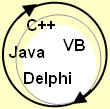Free eBook

Free ebook www.ebook88.com

Jogena's - eBook and eZine Directories - Get Listed Today!

Open Source Code

Open Source If you are also an Android Studio programmer, you can use full smartphone application projects. Projects contain full Java source code and all libraries and compile without errors. Projects contain all comments

AudioREG_ENG.ZIP >> - Audio Registrar Source Code

CryptoNote_password_OK.ZIP >> - Crypto Note Source Code

CryptoNOTE_AES.ZIP >> - AES Crypto Note Source Code

PhotoREGISTRAR >> - Photo Registrar with FTP Client

RSA Crypto Open Source Code:

RSA Crypto Project >> - RSA Keys, encoding, decodind

AES Crypto Project >> - AES Crypto Note Source Code
GNU GPL
Valery Shmelev

Android Studio Open Source

Android JAVA Code >>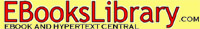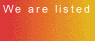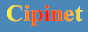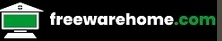Ebook. Manual on development of a logic game in Delphi is identical to the manual versions for VB, Java and C++. It would help you to master new programming languages in a short time. The manual includes Word document with step-by-step description of the process of program development, screenshots, procedure codes and 14 complete listings in Delphi (Borland Delphi Enterprice Version 5 Build 5.62) illustrating all stages of programming in Delphi-Application.     © by Valery V SHmeleff http://www.oflameron.com         Game algorithm description         Game is conducted on a game field, which is optional size field (limited only to display size or convenience of programming). Since later on we’ll describe the process of developing the game graphic and PDA-versions, we have chosen 8x5 for the game table.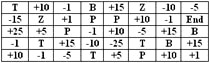All cells of the game field (table) are filled with symbols denoting the cell nominal values:          [-1,-5,-10,-15,-25] - deduct the indicated points from your account.     [1,5,10,15,25] - add the indicated points to your account.     [B] - deducts 200 points from your account.     [P] - adds 100 points to your account.     [T] - adds 500 points to your account.     [Z] - zeroes your account.     [END] - ends the game.          The player can perform only 2 actions on the game field:     1 – put “marker” on any cell of the game field bottom line only once at the game start (by clicking on the cell field).     2 – on putting the “marker”, the player can move it right or left along the game field bottom line by means of left and right arrows (keyboard button).     All cell nominal values to be “visited” by the player “marker” will be added to score. “Marker” is a cell highlighted in blue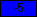. To put a marker, click on any cell of the game field bottom line. “Marker” may be put only once. Then it can be moved many times.     As soon as the player has put the marker, game starts. Game is conducted for time. A linear color indicator counts time intervals, at which all lines of game field are moved one line down. At that, a cell with a losing value may fall on the player “marker”. The player goal is to move the marker along winning cells, increase the score as possible, and watch the “marker” not to be “caught” by the cell from above with a losing nominal value (e.g., |Z|- score zeroing).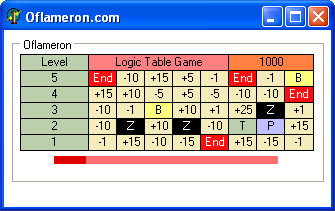Game is not as simple as it may seem. Its program has additional functions: acceleration and slowing down of game, changing winning cells frequency at the player large score. Game Oflameron may be realized in its static version, with no account of time. This version may have its benefits in the game process.          Game goal is to increase the score. Game (theoretically endless) may be stopped in the following situations: 1) the player “marker” falls to a cell with |End| nominal 2) the player score becomes negative 3) game has time limitation. Such flexibility of game algorithm allows to create a great number of its versions – with accumulation of “lives”, introduction of extra bonuses (cell nominal values), loading graphic design from the Internet, network versions for 2 players (static version) and paper version.              Android Studio Open Source
 GAME Programming          Create Project as Delphi5 Project1.pas and Form1, its size being 343х302. A GroupBox1: TGroupBox, StaticText1 – StaticText55 element is to be placed at the Form1. Where StaticText1 – StaticText6 is a Level column, StaticText7 – StaticText46 are nominal playing area cells, StaticText47-48 present control footing information. A lower color indicator is formed by StaticText49-StaticText55.          Number arrays correspond to a Level element column and a playing area.- StaticText1 – StaticText6 is a Level column          Create another StaticText56 to temporarily store a generated cell nominal and its colour attributes (highlighted at the figure).- temporarily store a generated cell nominal and its colour attributes          On the start of the game fill in playing area cells with random-number generator values. Random-number generator code:          Randomize;     StaticText7.Caption :=IntToStr(Random(20)); //Generates a random number = from 0 to 20          A complete Delphi project of this game development stage is in the file dlp1.zip (in Delphi-guide.zip)- Set lower color indicator size:          StaticText49.Height :=8;     StaticText50.Height :=8;     StaticText51.Height :=8;     StaticText52.Height :=8;     StaticText53.Height :=8;     StaticText54.Height :=8;     StaticText55.Height :=8;          StaticText49.Width :=32;     StaticText50.Width :=32;     StaticText51.Width :=32;     StaticText52.Width :=32;     StaticText53.Width :=32;     StaticText54.Width :=32;     StaticText55.Width :=32;          Use field array and i,j variable:          var     Form1: TForm1;     St: systemtime;     field: array[0..20, 0..20] of Integer; // Array for the playing area     i: byte;     j: byte;          Let us fill in game array cells field[0..20, 0..20] with values from the generator of random numbers:          function FieldFill : integer;     begin     {Let us fill cell array Field with random number generator values     }     Randomize;     for i := 0 to 8 do       for j := 0 to 6 do     field[i, j] :=Random(20);     end;          Now, procedure function Color_Chars of calculation of the nominal value of game area cell and its color attributes (cell background color and font color) shall be calculated basing on array element value. Example for processing of elements of the array field[0..20, 0..20] corresponding to cells of the bottom line of the game area (write them down to StaticText56):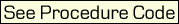Android Studio Open Source
 A complete Delphi project of this game development stage is in the file dlp2.zip (in Delphi-guide.zip)          As a result there appears a nominal copying function GameField_Fill from a responsible cell StaticText56, while a function Color_Chars is changed in the following way:Such variant of filling a playing area with START values (at the start of the game) cannot be considered as an optimal one. However it is very demonstrable for algorithm understanding.          To see how it works add another TForm1.StaticText56Click procedure          procedure TForm1.StaticText56Click(Sender: TObject);     begi     k := 0;     FieldFill();     GameField_Fill();     end;          Now, clicking the mouse to StaticText56 you can see if the cell nominals values and their colour attributes are changed correctly.          A complete Delphi project of this game development stage is in the file dlp3.zip (in Delphi-guide.zip)          Now develop Num_Move() procedure to overwrite playing area cells’ values and color attributes in a row-wise top-down way and to fill a playing area top row with new values (i.e., new cell values are input in the game from the top row). The procedure contains copying operators. You can develop a more optimal copying algorithm.          function Num_Move : integer;     begin     k := 0;     for j := 0 to 5 do       for i := 0 to 7 do         begin         field[i, j] := field[i, j + 1];         end;     GameField_Fill();     end;          To see how top-down copying values and cell attributes works, create a temporary click handling procedure at StaticText6 (cell Level 1):          procedure TForm1.StaticText6Click(Sender: TObject);     begin     Num_Move();     end;          A complete Delphi project of this game development stage is in the file dlp4.zip (in Delphi-guide.zip)          Now it necessary to add function Up_Str_App code to fill in the playing area top row with new values.          function Up_Str_App : integer;     begin     Randomize;     for i := 0 to 7 do       field[i, 5] :=Random(20);     end;          Call Up_Str_App from function Num_Move:          function Num_Move : integer;     begin     Up_Str_App();     k := 0;       for j := 0 to 5 do         for i := 0 to 7 do         begin           field[i, j] := field[i, j + 1];         end;     GameField_Fill();     end;         Android Studio Open Source
 Click the mouse to the StaticText6 ( Level     1     ) field and see how it works!          A complete Delphi project of this game development stage is in the file dlp5.zip (in Delphi-guide.zip)          Now it is necessary to write a handling code for player’s placing a game marker in any bottom row cell of the playing area.          Create a trigger firstset = 0          Trigger=0, if a marker has not been placed in the bottom row yet.          General code fragment that handles marker placing in the bottom row:          procedure TForm1.StaticText7Click(Sender: TObject);     begin     if firstset = 0 then Form1.StaticText7.Color := \$00FD1117; //Marker color     if firstset = 0 then markersave := 0; //StaticText7 cell     if firstset = 0 then firstset := 1; //Trigger. No use again     end;          procedure TForm1.StaticText8Click(Sender: TObject);     begin     if firstset = 0 then Form1.StaticText8.Color := \$00FD1117;     if firstset = 0 then markersave := 1; //StaticText8 cell     if firstset = 0 then firstset := 1; //Trigger. No use again     end;          procedure TForm1.StaticText9Click(Sender: TObject);     begin     if firstset = 0 then Form1.StaticText9.Color := \$00FD1117;     if firstset = 0 then markersave := 2; //StaticText9 cell     if firstset = 0 then firstset := 1; //Trigger. No use again     end;          procedure TForm1.StaticText10Click(Sender: TObject);     begin     if firstset = 0 then Form1.StaticText10.Color := \$00FD1117;     if firstset = 0 then markersave := 3; //StaticText10 cell     if firstset = 0 then firstset := 1; //Trigger. No use again     end;          procedure TForm1.StaticText11Click(Sender: TObject);     begin     if firstset = 0 then Form1.StaticText11.Color := \$00FD1117;     if firstset = 0 then markersave := 4; //StaticText11 cell     if firstset = 0 then firstset := 1; //Trigger. No use again     end;          procedure TForm1.StaticText12Click(Sender: TObject);     begin     if firstset = 0 then Form1.StaticText12.Color := \$00FD1117;     if firstset = 0 then markersave := 5; //StaticText12 cell     if firstset = 0 then firstset := 1; //Trigger. No use again     end;          procedure TForm1.StaticText13Click(Sender: TObject);     begin     if firstset = 0 then Form1.StaticText13.Color := \$00FD1117;     if firstset = 0 then markersave := 6; //StaticText13 cell     if firstset = 0 then firstset := 1; //Trigger. No use again     end;          procedure TForm1.StaticText14Click(Sender: TObject);     begin     if firstset = 0 then Form1.StaticText14.Color := \$00FD1117;     if firstset = 0 then markersave := 7; //StaticText14 cell     if firstset = 0 then firstset := 1; //Trigger. No use again     end;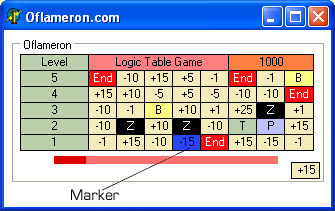The possibility of placing a marker on one cell only can be checked. Now it is necessary to provide a marker being saved (in terms of cell turning   blue  ) when shifting cells top – down.
 The function Marker_Reset : integer of marker colour restoring after playing area cells shifting top-down:          function Marker_Reset : integer     begin     if markersave = 0 then Form1.StaticText7.Color := \$00FD1117; //Marker color restoring     if markersave = 1 then Form1.StaticText8.Color := \$00FD1117;     if markersave = 2 then Form1.StaticText9.Color := \$00FD1117;     if markersave = 3 then Form1.StaticText10.Color := \$00FD1117;     if markersave = 4 then Form1.StaticText11.Color := \$00FD1117;     if markersave = 5 then Form1.StaticText12.Color := \$00FD1117;     if markersave = 6 then Form1.StaticText13.Color := \$00FD1117;     if markersave = 7 then Form1.StaticText14.Color := \$00FD1117;     end;          Now activate Marker_Reset() procedure to operate in a top-down shift procedure. In the bottom of function Num_Move procedure insert the following code (highlighted in red):          function Num_Move : integer;     begin     Up_Str_App(); //Fill in the top row with new nominals     k := 0; //Cells counter       for j := 0 to 5 do         for i := 0 to 7 do           begin           field[i, j] := field[i, j + 1];           end;     GameField_Fill();     Marker_Reset(); //Marker redraw     end;          A complete Delphi project of this game development stage is in the file dlp6.zip (in Delphi-guide.zip)          The procedure to save color attributes of all bottom row cells before placing the marker:          function Save_Color : integer;     savecolor :=Form1.StaticText7.Color; //Save bgcolor StaticText7     savecolor :=Form1.StaticText8.Color; //Save bgcolor StaticText8     savecolor :=Form1.StaticText9.Color; //Save bgcolor StaticText9     savecolor :=Form1.StaticText10.Color; //Save bgcolor StaticText10     savecolor :=Form1.StaticText11.Color; //Save bgcolor StaticText11     savecolor :=Form1.StaticText12.Color; //Save bgcolor StaticText12     savecolor :=Form1.StaticText13.Color; //Save bgcolor StaticText13     savecolor :=Form1.StaticText14.Color; //Save bgcolor StaticText14     end;          Write a score count function Set_Marker_Count when placing a marker in the beginning of the game.          function Set_Marker_Count : integer;     begin       if n = 0 then       begin       If markersave = 0 Then et := field[0, 0];       If markersave = 1 Then et := field[1, 0];       If markersave = 2 Then et := field[2, 0];       If markersave = 3 Then et := field[3, 0];       If markersave = 4 Then et := field[4, 0];       If markersave = 5 Then et := field[5, 0];       If markersave = 6 Then et := field[6, 0];       If markersave = 7 Then et := field[7, 0];     Form1.Caption := IntToStr(markersave);       end;     //Print count       if et = 0 then Form1.StaticText48.Caption := '+1';       if et = 1 then Form1.StaticText48.Caption := '-1';       if et = 2 then Form1.StaticText48.Caption := '+5';       if et = 3 then Form1.StaticText48.Caption := '-5';       if et = 4 then Form1.StaticText48.Caption := '+10';       if et = 5 then Form1.StaticText48.Caption := '-10';       if et = 6 then Form1.StaticText48.Caption := '+15';       if et = 7 then Form1.StaticText48.Caption := '-15';       if et = 8 then Form1.StaticText48.Caption := '+25';       if et = 9 then Form1.StaticText48.Caption := '+500';       if et = 10 then Form1.StaticText48.Caption := '+100';       if et = 11 then Form1.StaticText48.Caption := '-200';       if et = 12 then Form1.StaticText48.Caption := '0';       if et = 13 then Form1.StaticText48.Caption := '0';       if et = 14 then Form1.StaticText48.Caption := 'End';       if et = 15 then Form1.StaticText48.Caption := '-10';       if et = 16 then Form1.StaticText48.Caption := '-5';       if et = 17 then Form1.StaticText48.Caption := '-1';       if et = 18 then Form1.StaticText48.Caption := '+1';       if et = 19 then Form1.StaticText48.Caption := '+5';       if et = 20 then Form1.StaticText48.Caption := '-25';       n := 1; //No use again     end;          Now attach a score count function Set_Marker_Count in each of click procedures for bottom row cells:
 procedure TForm1.StaticText7Click(Sender: TObject);     begin     if firstset = 0 then Form1.StaticText7.Color := \$00FD1117;     if firstset = 0 then markersave := 0; //markersave := 0 - for StaticText7     if firstset = 0 then firstset := 1; //No use again     Set_Marker_Count();     end;          procedure TForm1.StaticText8Click(Sender: TObject);     begin     if firstset = 0 then Form1.StaticText8.Color := \$00FD1117;     if firstset = 0 then markersave := 1; //markersave := 1 - for StaticText8     if firstset = 0 then firstset := 1; //No use again     Set_Marker_Count();     end;          procedure TForm1.StaticText9Click(Sender: TObject);     begin     if firstset = 0 then Form1.StaticText9.Color := \$00FD1117;     if firstset = 0 then markersave := 2; //markersave := 2 - for StaticText9     if firstset = 0 then firstset := 1; //No use again     Set_Marker_Count();     end;          procedure TForm1.StaticText10Click(Sender: TObject);     begin     if firstset = 0 then Form1.StaticText10.Color := \$00FD1117;     if firstset = 0 then markersave := 3; //markersave := 3 - for StaticText10     if firstset = 0 then firstset := 1; //No use again     Set_Marker_Count();     end;          procedure TForm1.StaticText11Click(Sender: TObject);     begin     if firstset = 0 then Form1.StaticText11.Color := \$00FD1117;     if firstset = 0 then markersave := 4; //markersave := 4 - for StaticText11     if firstset = 0 then firstset := 1; //No use again     Set_Marker_Count();     end;          procedure TForm1.StaticText12Click(Sender: TObject);     begin     if firstset = 0 then Form1.StaticText12.Color := \$00FD1117;     if firstset = 0 then markersave := 5; //markersave := 5 - for StaticText12     if firstset = 0 then firstset := 1; //No use again     Set_Marker_Count();     end;          procedure TForm1.StaticText13Click(Sender: TObject);     begin     if firstset = 0 then Form1.StaticText13.Color := \$00FD1117;     if firstset = 0 then markersave := 6; //markersave := 6 - for StaticText13     if firstset = 0 then firstset := 1; //No use again     Set_Marker_Count();     end;          procedure TForm1.StaticText14Click(Sender: TObject);     begin     if firstset = 0 then Form1.StaticText14.Color := \$00FD1117;     if firstset = 0 then markersave := 7; //markersave := 7 - for StaticText14     if firstset = 0 then firstset := 1; //No use again     Set_Marker_Count();     end;          A complete Delphi project of this game development stage is in the file dlp7.zip (in Delphi-guide.zip)          Rewrite function Set_Marker_Count          function Set_Marker_Count : integer;     begin       if n = 0 then       begin       If markersave = 0 Then et := field[0, 0];       If markersave = 1 Then et := field[1, 0];       If markersave = 2 Then et := field[2, 0];       If markersave = 3 Then et := field[3, 0];       If markersave = 4 Then et := field[4, 0];       If markersave = 5 Then et := field[5, 0];       If markersave = 6 Then et := field[6, 0];       If markersave = 7 Then et := field[7, 0];     Form1.Caption := IntToStr(markersave);       end;     //Print count            if n = 0 then       begin       tk := StrToInt(Form1.StaticText48.Caption); //Count to digit      See details ::       //StrToInt - converts an Integer string, such as '123' into an Integer       if et = 0 then tk := tk +1; //Cell nominal       if et = 0 then Form1.StaticText48.Caption := IntToStr(tk); //StaticText48 - counter cell       if et = 1 then tk := tk -1; //Cell nominal       if et = 1 then Form1.StaticText48.Caption := IntToStr(tk);       if et = 2 then tk := tk +5; // ...       if et = 2 then Form1.StaticText48.Caption := IntToStr(tk);       if et = 3 then tk := tk -5;       if et = 3 then Form1.StaticText48.Caption := IntToStr(tk);       if et = 4 then tk := tk +10;       if et = 4 then Form1.StaticText48.Caption := IntToStr(tk);       if et = 5 then tk := tk -10;       if et = 5 then Form1.StaticText48.Caption := IntToStr(tk);       if et = 6 then tk := tk +15;       if et = 6 then Form1.StaticText48.Caption := IntToStr(tk);       if et = 7 then tk := tk -15;       if et = 7 then Form1.StaticText48.Caption := IntToStr(tk);       if et = 8 then tk := tk +25;       if et = 8 then Form1.StaticText48.Caption := IntToStr(tk);       if et = 9 then tk := tk +500; //Nominal [T]       if et = 9 then Form1.StaticText48.Caption := IntToStr(tk);       if et = 10 then tk := tk +100; //Nominal [P]       if et = 10 then Form1.StaticText48.Caption := IntToStr(tk);       if et = 11 then tk := tk -200; //Nominal [B]       if et = 11 then Form1.StaticText48.Caption := IntToStr(tk);              if et = 12 then Form1.StaticText48.Caption := '0'; //Nominal [Z]       if et = 13 then Form1.StaticText48.Caption := '0'; //Nominal [Z]       if et = 14 then Form1.StaticText48.Caption := 'End'; //End game       if et = 14 then Application.Terminate; //End game              if et = 15 then tk := tk -10;       if et = 15 then Form1.StaticText48.Caption := IntToStr(tk);       if et = 16 then tk := tk -5;       if et = 16 then Form1.StaticText48.Caption := IntToStr(tk);       if et = 17 then tk := tk -1;       if et = 17 then Form1.StaticText48.Caption := IntToStr(tk);       if et = 18 then tk := tk +1;       if et = 18 then Form1.StaticText48.Caption := IntToStr(tk);       if et = 19 then tk := tk +5;       if et = 19 then Form1.StaticText48.Caption := IntToStr(tk);       if et = 20 then tk := tk -25;       if et = 20 then Form1.StaticText48.Caption := IntToStr(tk);       n := 1; //No use again. Marker already set     end;     end;
 Now write a score counting procedure at cells’ shifting top-down. Procedure looks like extremely simple:          function Dn_Count : integer;     begin     n := 0; //Temporary enable Set_Marker_Count     Set_Marker_Count();     end;          To demonstrate the procedure operation, insert the procedure call in a top-down cell row shifting procedure TForm1.StaticText6Click          procedure TForm1.StaticText6Click(Sender: TObject);     begin     Num_Move();     Dn_Count();     end;          A complete Delphi project of this game development stage is in the file dlp8.zip (in Delphi-guide.zip)          Create a procedure of linear color indicatoroperation. Add Timer1 to the form and enable it at once.          procedure TForm1.Timer1Timer(Sender: TObject);     begin     IndLent := IndLent + 1;     if IndLent = 9 then Timer1.Interval := 500; //Timer interval recovery       if IndLent = 9 then IndLent := 0;     Print_Ind(); //Draw linear color indicator     end;          Procedure of linear color indicator operation:          function Print_Ind : integer;     begin     if IndLent = 1 then Form1.StaticText55.Visible := False;     if IndLent = 2 then Form1.StaticText54.Visible := False;     if IndLent = 3 then Form1.StaticText53.Visible := False;     if IndLent = 4 then Form1.StaticText52.Visible := False;     if IndLent = 5 then Form1.StaticText51.Visible := False;     if IndLent = 6 then Form1.StaticText50.Visible := False;     if IndLent = 7 then Form1.StaticText49.Visible := False;     if IndLent = 7 then Form1.Timer1.Interval := 100;          if IndLent = 7 then //Draw full linear color indicator     begin       Form1.StaticText55.Visible := True;       Form1.StaticText54.Visible := True;       Form1.StaticText53.Visible := True;       Form1.StaticText52.Visible := True;       Form1.StaticText51.Visible := True;       Form1.StaticText50.Visible := True;       Form1.StaticText49.Visible := True;       end;     end;          You can see how the indicator works.          A complete Delphi project of this game development stage is in the file dlp9.zip (in Delphi-guide.zip)          Now you should disable the timer and enable it after the market has been placed in a playing area bottom row. Insert timer enabling in a marker placing procedure in a playing area bottom row (to be more exact – in a score counting procedure after marker placing Set_Marker_Count(), since the procedure is common for all cells of a lower level).     At the end of the procedure Set_Marker_Count() there is a line:          Form1.Timer1.Enabled := True; //Timer1 is ON          When a linear color indicator disappears completely, it is necessary to shift down all rows with playing area cells (TForm1.StaticText6Click procedure) to the marker, count scores and fill in a playing area top row with new nominal values.          TForm1.Timer1Timer operation procedure looks like the following way:          procedure TForm1.Timer1Timer(Sender: TObject);     begin     IndLent := IndLent + 1;     if IndLent = 9 then Num_Move();//Move game levels (Rows)     if IndLent = 9 then Dn_Count();     if IndLent = 9 then Timer1.Interval := 500; //Restore interval     if IndLent = 9 then IndLent := 0;     Print_Ind(); //Draw indicator     End If     end;          Now it is necessary to write handling of pressing down the key and marker’s moving to the right / left along the bottom row of playing area cells.          procedure TForm1.FormKeyDown(Sender: TObject; var Key: Word; Shift: TShiftState);     //Handling of pressing right and left arrow keys     begin     Form1.Caption := IntToStr(Key); //Print key kode for debugging purposes     end;          markersave variable shows a unique playing area bottom row cell that contains a marker. Write a procedure of marker moving to the left / right along the bottom row:          function Move_Point_Left : integer; //Move marker to left     begin       if markersave = 0 then //If the most left cell of the bottom line       begin       markersave := 7; //More to the left of the most left cell - the MOST RIGHT (cyclic transition)       firstset := 0; //Temporarily to resolve drawing a marker       Form1.StaticText7.Color := savecolor; //To restore color of a background of a cell where the marker was       //As in TForm1.StaticText14Click procedure. Set marker       if firstset = 0 then Save_Color(); //Save color       if firstset = 0 then Form1.StaticText14.Color := \$00FD1117;       if firstset = 0 then markersave := 7;       if firstset = 0 then firstset := 1;       Set_Marker_Count();       vt := markersave; //Save markersave       markersave := 10; //Disable misoperation (value 10 does not correspond to any cell)       end;     markersave := vt; //Restore markersave     n := 0;     Set_Marker_Count();     end;          A complete Delphi project of this game development stage is in the file dlp10.zip (in Delphi-guide.zip)
 Full code for function Move_Point_Left          function Move_Point_Left : integer;     begin     if markersave = 0 then //If the most Left cell of the bottom line     begin       markersave := 7;       firstset := 0; //Temporarily to resolve drawing a marker       Form1.StaticText7.Color := savecolor; //Restore bgcolor         if firstset = 0 then Save_Color(); //Save color         if firstset = 0 then Form1.StaticText14.Color := \$00FD1117;         if firstset = 0 then markersave := 7;         if firstset = 0 then firstset := 1;         Set_Marker_Count();       vt := markersave; //Save markersave       markersave := 10; //Disable misoperation (value 10 does not correspond to any cell)     end;          if markersave = 1 then //If the StaticText8 cell of the bottom line     begin       markersave := 0;       firstset := 0; //Temporarily to resolve drawing a marker       Form1.StaticText8.Color := savecolor; //Restore bgcolor         if firstset = 0 then Save_Color(); //Save color         if firstset = 0 then Form1.StaticText7.Color := \$00FD1117;         if firstset = 0 then markersave := 0;         if firstset = 0 then firstset := 1;         Set_Marker_Count();       vt := markersave; //Save markersave       markersave := 10; //Disable misoperation (value 10 does not correspond to any cell)     end;          if markersave = 2 then //If the StaticText9 cell of the bottom line     begin       markersave := 1;       firstset := 0; //Temporarily to resolve drawing a marker       Form1.StaticText9.Color := savecolor; //Restore bgcolor         if firstset = 0 then Save_Color(); //Save color         if firstset = 0 then Form1.StaticText8.Color := \$00FD1117;         if firstset = 0 then markersave := 1;         if firstset = 0 then firstset := 1;         Set_Marker_Count();       vt := markersave; //Save markersave       markersave := 10; //Disable misoperation (value 10 does not correspond to any cell)     end;          if markersave = 3 then //If the StaticText10 cell of the bottom line     begin       markersave := 2;       firstset := 0; //Temporarily to resolve drawing a marker       Form1.StaticText10.Color := savecolor; //Restore bgcolor         if firstset = 0 then Save_Color(); //Save color         if firstset = 0 then Form1.StaticText9.Color := \$00FD1117;         if firstset = 0 then markersave := 2;         if firstset = 0 then firstset := 1;         Set_Marker_Count();       vt := markersave; //Save markersave       markersave := 10; //Disable misoperation (value 10 does not correspond to any cell)     end;               if markersave = 4 then //If the StaticText11 cell of the bottom line     begin       markersave := 3;       firstset := 0; //Temporarily to resolve drawing a marker       Form1.StaticText11.Color := savecolor; //Restore bgcolor         if firstset = 0 then Save_Color(); //Save color         if firstset = 0 then Form1.StaticText10.Color := \$00FD1117;         if firstset = 0 then markersave := 3;         if firstset = 0 then firstset := 1;         Set_Marker_Count();       vt := markersave; //Save markersave       markersave := 10; //Disable misoperation (value 10 does not correspond to any cell)     end;          if markersave = 5 then //If the StaticText12 cell of the bottom line     begin       markersave := 4;       firstset := 0; //Temporarily to resolve drawing a marker       Form1.StaticText12.Color := savecolor; //Restore bgcolor         if firstset = 0 then Save_Color(); //Save color         if firstset = 0 then Form1.StaticText11.Color := \$00FD1117;         if firstset = 0 then markersave := 4;         if firstset = 0 then firstset := 1;         Set_Marker_Count();       vt := markersave; //Save markersave       markersave := 10; //Disable misoperation (value 10 does not correspond to any cell)     end;          if markersave = 6 then //If the StaticText13 cell of the bottom line     begin       markersave := 5;       firstset := 0; //Temporarily to resolve drawing a marker       Form1.StaticText13.Color := savecolor; //Restore bgcolor         if firstset = 0 then Save_Color(); //Save color         if firstset = 0 then Form1.StaticText12.Color := \$00FD1117;         if firstset = 0 then markersave := 5;         if firstset = 0 then firstset := 1;         Set_Marker_Count();       vt := markersave; //Save markersave       markersave := 10; //Disable misoperation (value 10 does not correspond to any cell)     end;          if markersave = 7 then //If the StaticText14 cell - most Right cell of the bottom line     begin       markersave := 6;       firstset := 0; //Temporarily to resolve drawing a marker       Form1.StaticText14.Color := savecolor; //Restore bgcolor         if firstset = 0 then Save_Color(); //Save color         if firstset = 0 then Form1.StaticText13.Color := \$00FD1117;         if firstset = 0 then markersave := 6;         if firstset = 0 then firstset := 1;         Set_Marker_Count();       vt := markersave; //Save markersave       markersave := 10; //Disable misoperation (value 10 does not correspond to any cell)     end;          markersave := vt; //Restore markersave     n := 0;     Set_Marker_Count();     end;          Moving to Right is similar.

Handling of pressing down the key:

procedure TForm1.FormKeyDown(Sender: TObject; var Key: Word; Shift: TShiftState);
begin
Form1.Caption := IntToStr(Key);
if Key = 37 then Move_Point_Left();
//Move to Left
if Key = 39 then Move_Point_Right();
//Move to Right
end;

A complete Delphi project of this game development stage is in the file dlp11.zip (in
Delphi-guide.zip)

Now it is necessary to create only one procedure – a procedure of shifting the numbering of current gaming levels:

function Level_Count : integer;
begin
lvl := StrToInt(Form1.StaticText6.Caption);
lvl := lvl + 1;
Form1.StaticText6.Caption := IntToStr(lvl);

lvl := StrToInt(Form1.StaticText5.Caption);
lvl := lvl + 1;
Form1.StaticText5.Caption := IntToStr(lvl);

lvl := StrToInt(Form1.StaticText4.Caption);
lvl := lvl + 1;
Form1.StaticText4.Caption := IntToStr(lvl);

lvl := StrToInt(Form1.StaticText3.Caption);
lvl := lvl + 1;
Form1.StaticText3.Caption := IntToStr(lvl);

lvl := StrToInt(Form1.StaticText2.Caption);
lvl := lvl + 1;
Form1.StaticText2.Caption := IntToStr(lvl);

end;

Attach a shifting procedure to the operations, for example:

function Dn_Count : integer;
begin
n := 0;
Set_Marker_Count();
Level_Count(); //Shifting the numbering of current gaming levels
end;

A complete Delphi project of this game development stage is in the file dlp12.zip (in
Delphi-guide.zip)

Crosswalk Directory:
Over 18,000 wholesome, family friendly, Christian websites.

 Rate Our Ebook - Select -Excellent!Very GoodGoodFairPoor @ eLibrary

 Now, this is a demonstrative moment: all software-realization procedures of the game Oflameron are ready. In other words, you have created a completely operable logical gaming program in Delphi 5.     It is still early to introduce it as a commercial product as it still contains small faults. Removing such faults and configuring the game as a commercial product are dealt in the 2nd & 3d parts of the guidelines.          Logical game OFLAMERON (www.oflameron.ru) has several variants, such as tabular, card, cycle card, paper sheet tabular. Software versions of the game contain bigger scaling capabilities: playing area dimensions can be changed, as well as cells’ / cards’ nominal quantity, game branching types can; PDA /cellular versions can be created etc.Warning! Free for any nonecommercial use and:     - the name of the game, game algorithm and the face values (nominals) of cards should be kept;     - the game, manual, code should contain the following text without changes:     " © by Valery V SHmeleff / Moscow,Russia 1996-2005 http://www.oflameron.com "          ALEX     PYTHON Machine learning     This is a project of Me. Also, let me say in advance that most of files in this repository are stored here just as the first example of our work. It was written using numpy and pytorch and this file doesn't affect our main program.          Here are given three folders: rnn, selam and tasks. Let's define what each of them is dedicated for:          rnn - this folder store the major part of our work, thus you can find code of the NN (and their saved states) and the important file with settings there     selam - store the bash script which allow you to quickly generate data to train NN using SELAM generator     tasks - mainly store .sbatch files which are used to run programs using SLURM          PYTHON Programmer >>          PYTHON and SQL Programmer